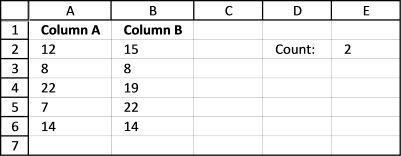Author: Oscar Cronquist Article last updated on August 19, 2017

Hi Oscar,Need a formula to count identical numbers in two columns but items must be in same row (position).

12 15
8 8 good count 1
22 19
7 22 for 22 not count cause is not in same row
14 14 good count 2Array formula in cell E2:

=SUM((A2:A6=B2:B6)*1)

How to enter an array formula

1. Select cell E2
2. Paste the formula in formula bar
3. Press and hold CTRL + SHIFT simultaneously
4. Press Enter
5. Release all keys

Your formula now begins and ends with a curly bracket, if you did it right.

Like this {=SUM((A2:A6=B2:B6)*1)}

### Explaining formula

Step 1 - Compare values in column A with column B

A2:A6=B2:B6

becomes

{12;8;22;7;14}={15;8;19;22;14}

and returns

{FALSE;TRUE;FALSE;FALSE;TRUE}

Step 2 - Multiply boolean values with 1

To be able to sum the values in this array {FALSE;TRUE;FALSE;FALSE;TRUE} we need to convert them to 0 and 1. FALSE = 0 and TRUE = 1.

(A2:A6=B2:B6)*1

becomes

({FALSE;TRUE;FALSE;FALSE;TRUE})*1

and returns

{0;1;0;0;1}

Step 3 - Sum values in array

SUM((A2:A6=B2:B6)*1)

becomes

SUM({0;1;0;0;1})

and returns 2.

This formula also works with text values, to remove blanks before and after use TRIM function.

=SUM((TRIM(A2:A6)=TRIM(B2:B6))*1)

### Download excel *.xlsx file

Count identical numbers in two columns but items must be in same row.xlsx